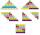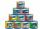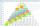# Chairs

Determine the number of seats in the seventh row and ninth row, if 3rd row has 14 seats and in every next row of seats has five more than the previous row.

Result

a7 =  34
a9 =  44

#### Solution:Leave us a comment of example and its solution (i.e. if it is still somewhat unclear...):

Showing 0 comments:Be the first to comment!#### To solve this example are needed these knowledge from mathematics:

Do you have a linear equation or system of equations and looking for its solution? Or do you have quadratic equation?

## Next similar examples:

1. AS sequenceIn an arithmetic sequence is given the difference d = -3 and a71 = 455. a) Determine the value of a62 b) Determine the sum of 71 members.
2. AP - simpleDetermine the first nine elements of sequence if a10 = -1 and d = 4
3. Fifth memberDetermine the fifth member of the arithmetic progression, if the sum of the second and fifth members equal to 73, and difference d = 7.
4. Third memberDetermine the third member of the AP if a4=93, d=7.5.
5. Determine APDetermine the difference of the arithmetic progression if a3 = 7, and a4 + a5 = 71
6. Theorem proveWe want to prove the sentense: If the natural number n is divisible by six, then n is divisible by three. From what assumption we started?
7. SeatsSeats in the sport hall are organized so that each subsequent row has five more seats. First has 10 seats. How many seats are: a) in the eighth row b) in the eighteenth row
8. SequenceBetween numbers 1 and 53 insert n members of the arithmetic sequence that its sum is 702.
9. DigitsShow that if x, y, z are 3 consecutive nonzero digits, zyx-xyz = 198, where zyx and xyz are three-digit numbers created from x, y, z.
10. Holidays - on poolChildren's tickets to the swimming pool stands x € for an adult is € 2 more expensive. There was m children in the swimming pool and adults three times less. How many euros make treasurer for pool entry?
11. SequenceWrite the first 7 members of an arithmetic sequence: a1=-3, d=6.
12. CansHow many cans must be put in the bottom row if we want 182 cans arrange in 13 rows above so that each subsequent row has always been one tin less? How many cans will be in the top row?
13. Difference AP 4Calculate the difference of the AP if a1 = 0.5, a2 + a3 = -1.1
14. Sequence 2Write the first 5 members of an arithmetic sequence a11=-14, d=-1
15. Sequence 3Write the first 5 members of an arithmetic sequence: a4=-35, a11=-105.
16. SequenceWrite the first 6 members of these sequence: a1 = 5 a2 = 7 an+2 = an+1 +2 an
17. Arithmetic progressionIn some AP applies: 5a2 + 7a5 = 90 s3 = 12 Find the first member a =? and difference d = ?